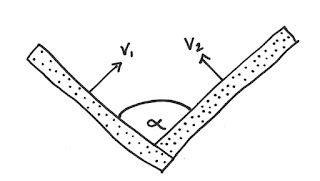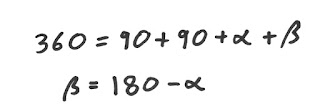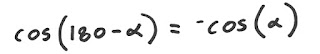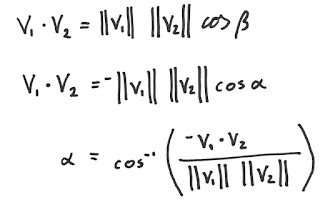## Friday, September 23, 2016

### I hope you find this blog enjoyable and helpful. Support it with a small donation.

Just a quick one today.  Up until recently I had never heard of the term dihedral angle.  It sounds complicated but it's something you already know.  If you have two surfaces in spaces that meet, they will form a line. The angle between the two surfaces at this intersection is called the dihedral angle.

I had recently been wondering about how to calculate the angle between two surfaces and decided to work through the problem myself.  First of all you need to describe the surfaces and I thought that the best way to do this was by supplying vectors normal to each surface, v1 and v2.Angle between two surfaces

By simplifying the geometry and extending the normal vectors until they intersect you can start to see how to solve the problem.Extended surface normal vectors

You can also derive the following formula from the geometry.Relation between alpha and beta

From this you can see that the cosine of beta is equal to the negative cosine of alpha.Simplified cosine term

By taking the dot product of the vectors v1 and v2 you can quickly derive an expression for the angle alpha.Expression for alpha

Great!  Problem solved.  Or is it?  The inverse cosine function only returns values between 0 and 180. This means you will never get an answer greater than 180 degrees, meaning you can't have convex angles.  The image below demonstrates why.  Given the vectors v1 and v2 to define the surfaces in the first part of the image below you would quickly run into trouble.  You can see in the lower part of the image that the same two vectors can be used to define a different geometry with a concave angle.Ambiguity between vectors

As the equation for alpha above doesn't change if the vectors v1 and v2 are swapped the two geometries above will give the same angle.  There are ways to work around this problem, you just need to be aware of the geometry you're working with.  I'm still having trouble getting my head around this.  The order the angles are given is important and would allow you to work out if the angle is concave or convex, but when generalising this to 3 dimensions you have trouble depending on what angle you look at joint.  I'm falling asleep typing this with pictures of vectors dancing around my head.  Maybe a fresh set of eyes will help.